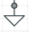# Linear Algebra - Analysis of purely resistive DC circuit

• Engineering
YoshiMoshi

## Homework Statement

I'm trying to solve this circuit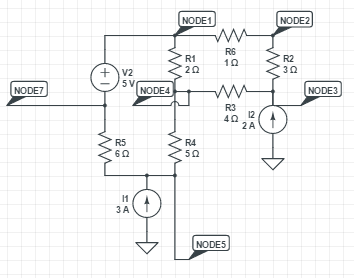when I try to simulate and run a dc sweep i get that it can't be solved. When I try to find the answer using linear algebra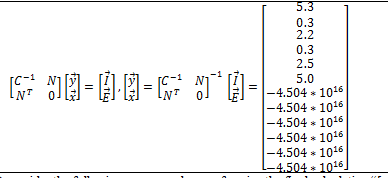I get this answer after I throw it into MATLAB with the warning

“[w]arning: Matrix is close to singular or badly scaled. Results may be inaccurate. RCOND = 2.312965e-18”.

## The Attempt at a Solution

I don't understand what I'm doing wrong. Thanks for any help.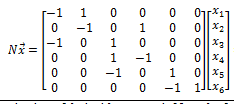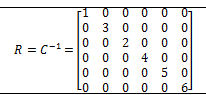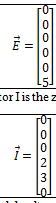I'm stuck in the linear algebra part and not the electrical engineering aspect so i figured i'd post it here.

thanks.

Mentor
In the simulation, can you try to debug it by running separate simulations with one or two of the power sources disabled? Short the voltage source and open a current source to see if that simulation runs. Try each of the 3 simulations with only one power source enabled. Then try combinations of two power sources enabled. Offhand, I don't see why the circuit isn't resolving. You could also try forcing some initial conditions to see if that helps with convergence to the DC operating points...

Mentor
BTW, are you able to just solve it by hand using the KCL equations?

Staff Emeritus
Homework Helper
i don't think that circuit is consistent with KCL. The current sources inject 5 A into the circuit, but there's no way for that current to leave the circuit.

•berkeman
YoshiMoshi
Vela - So I have to add in a ground at node 7 so that there's a place for the 5 amps to leave the circuit?

The simulation ran with the ground at node 7.

However won't the incidence matrix be the same? MATLAB would still give me that weird error. I think I'm doing something wrong with the linear algebra?

POSTING NEW PICTURE IN THIS POST OF CIRCUIT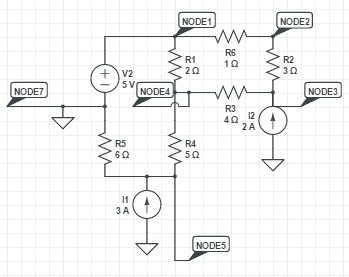YoshiMoshi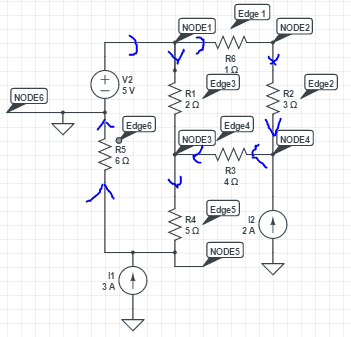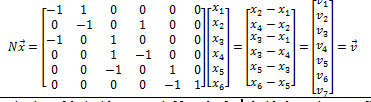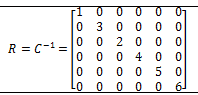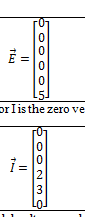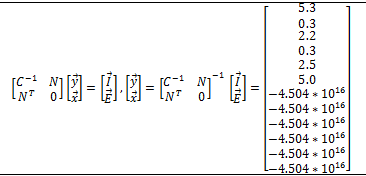I don't understand what I'm doing wrong or understand why MATLAB is giving me an error

>> N=[-1,1,0,0,0,0;0,-1,0,1,0,0;-1,0,1,0,0,0;0,0,1,-1,0,0;0,0,-1,0,1,0;0,0,0,0,-1,1];
>> R=[1,0,0,0,0,0;0,3,0,0,0,0;0,0,2,0,0,0;0,0,0,4,0,0;0,0,0,0,5,0;0,0,0,0,0,6];
>> E=[0;0;0;0;0;5];
>> I=[0;0;0;2;3;0];
>> A=[0,0,0,0,0,0;0,0,0,0,0,0;0,0,0,0,0,0;0,0,0,0,0,0;0,0,0,0,0,0;0,0,0,0,0,0];
>> inv([R,N;N',A])[I;E]
inv([R,N;N',A])[I;E]

Error: Unbalanced or unexpected parenthesis or
bracket.

>> inv([R,N;N',A])*[I;E]
Warning: Matrix is close to singular or badly
scaled. Results may be inaccurate. RCOND =
2.312965e-18.

ans =

1.0e+16 *

0.0000
0.0000
0.0000
0.0000
0.0000
0.0000
-4.5036
-4.5036
-4.5036
-4.5036
-4.5036
-4.5036

My simulator says that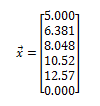I don't see what I'm doing wrong in MATLAB =(

Staff Emeritus
Homework Helper
Vela - So I have to add in a ground at node 7 so that there's a place for the 5 amps to leave the circuit?
Did you have a reason to choose node 7 in particular? It seems kind of arbitrary.

YoshiMoshi
No reason. I'm just use to putting the ground at the negative lead of the voltage source. It shouldn't matter right where we put the ground? I was able to simulate the circuit and got a solution.

I think MATLAB gets the error when it performs the following calculation
>>([R,N;N',A])^(-1)

Indeed just putting this into MATLAB returns the same error (except with different numbers of course). I don't see what's wrong with the calculation. I'm trying to get it to take the inverse of a 12x12 matrix split up in block form so it shouldn't have a problem.

I even try this
>>F=([R,N;N',A])
it does indeed return a matrix that has 12 collumns and 12 rows. I try this calculation
>>inv(F)
and I get the same error
"Matrix is close to singular or badly scaled. REsults may be inaccurate..."

I don't see what's wrong. =(

YoshiMoshi
Oh... so MATLAB thinks the matrix is singular? I just tried det(F) and it returned
1.1102*10^(-15) so even though it's not singular MATLAB thinks it is because the determinant is almost zero?

Gold Member
Oh... so MATLAB thinks the matrix is singular? I just tried det(F) and it returned
1.1102*10^(-15) so even though it's not singular MATLAB thinks it is because the determinant is almost zero?

Your incidence matrix is singular. Perhaps when you ground a node, the grounded node should vanish from the incidence matrix?

Mentor
@vela had it right in post #4. There are only two ground connections in the circuit and both are affixed to current sources. Ground symbols in this case are really just labels used in place of wiring the common connections together. If you replace the symbols with wires: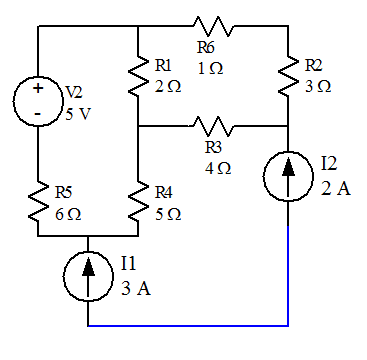You can see that there are two problems:

1. 5 A are injected into the circuit with no return path (might make an excellent Van DeGraff generator replacement!).
2. Two current sources with different current values are in series. Flash! Bang! Smoke!YoshiMoshi
Hi Gnelli,

Thanks for your response. Yeah I think your right but I find it strange that the circuit would run in the simulator when it is indeed two current sources in parallel. But is it really in parallel if they are separated by resistors? I have redrawn the circuit and have tried to use MATLAB again by modifying the circuit to remove one of the current sources and to have the only ground in the circuit be the one at the other end of the current source. MATLAB still doesn't seem to like it =(. I don't see what I'm doing wrong. Thanks for any help.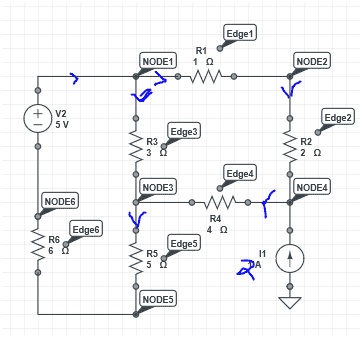Equations on modified circuit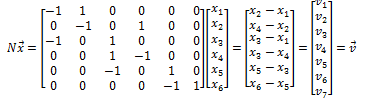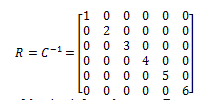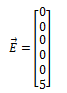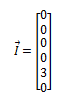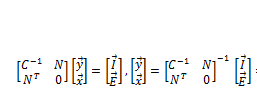putting my equations into MATLAB provides with the following answer as well as a warning that the matrix is singular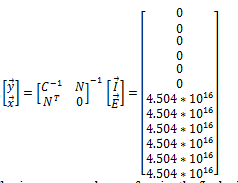MATLAB commands

>> N=[-1,1,0,0,0,0;0,-1,0,1,0,0;-1,0,1,0,0,0;0,0,1,-1,0,0;0,0,-1,0,1,0;0,0,0,0,-1,1];
>> R=[1,0,0,0,0,0;0,2,0,0,0,0;0,0,3,0,0,0;0,0,0,4,0,0;0,0,0,0,5,0;0,0,0,0,0,6];
>> A=[0,0,0,0,0,0;0,0,0,0,0,0;0,0,0,0,0,0;0,0,0,0,0,0;0,0,0,0,0,0;0,0,0,0,0,0];
>> E=[0;0;0;0;0;5];
>> I=[0;0;0;0;3;0];
>> inv([R,N;N',A])*[I;E]
Warning: Matrix is close to singular or badly
scaled. Results may be inaccurate. RCOND =
2.312965e-18.

ans =

1.0e+16 *

0
0
0
0
0.0000
0.0000
4.5036
4.5036
4.5036
4.5036
4.5036
4.5036

and when I try to take the determinant of the matrix [R,N;N',A] I get

-1.1102e-15

which may be to close to zero and very close to singular causing the error that I'm getting in MATLAB.

When I check the values in my simulator it tells me this time that it's unable to get the solution but I'm not sure what's wrong with my circuit. Thanks for any help!

Gold Member
Where is the return path for the current source?YoshiMoshi
I'm not exactly sure what you are talking about by return path to the current source or even what one would like. Can you help? I'm not sure what you are describing.

Gold Member
I1, if it is a current source then their are electrons flowing out of one of its terminals. What route do they take to get back in the other terminal?

YoshiMoshi
So your saying that I need to add a ground to the circuit? is node 6 ok to place the ground? This would allow the circuit to be simulated I think?

Also I think my equations would still be the same and MATLAB would still give me an error? I'm not sure it would effect the linear algebra equations.

Thanks for all of your help!

Gold Member
Put a ground anywhere you wish. As it stands, the lower end of I1 is goimg nowhere, as far as the ckt. is concerned it is floating.

YoshiMoshi
Ok sounds good, but wouldn't my linear equations all be the same and MATLAB still provide me with an error?

I think I'll place it at node 6 since I'm used to having a ground at the negative end of the battery terminal mostly.

I just don't see though does this effect the equations at all?

Gold Member
Well, if you try using KCL you'll find that you can't solve for the lower end of I1.

YoshiMoshi
You mean the current coming out of the battery?

Gold Member
I mean the current from the current source, that thing labeled I1.

YoshiMoshi
Oh so your talking about the lower part of the current source? I thought that there was no current comming from the ground to the current source and that the current is generated from the current source? At node 4 I have current coming into the node from R2 current going into the node from I1 and current leaving the node into R4?

I was able to simulate the circuit after placing a ground at node 6. However I was unable to place the pinpointer on the wire that connects the current source to the ground. I thought that there was 0 current at this point in the wire and that there was the 2A comming out of the current source?

Sorry I guess I never thought about that part of the wire.

Although I do remember learning something about avoiding applying KCL at ground

Gold Member
I thought that there was no current comming from the ground to the current source and that the current is generated from the current source?
I thought that there was 0 current at this point in the wire and that there was the 2A comming out of the current source?
That seems to be the basis of the problem.
You may recall that for current to flow there must be a closed path from the source, thru a load, back into the source. That's why devices have at least two terminals, one for electrons to leave from and one for electrons to return to. The present circuit does not have a way for electrons to get back to the current source lower terminal. It is as if you connected a light bulb to only one terminal of a battery, the bulb won't light because there is no return path.

YoshiMoshi
Ok I think it makes since now. So if I update the circuit with a ground at node 6, than the ground acts a return bath to the current source? Like so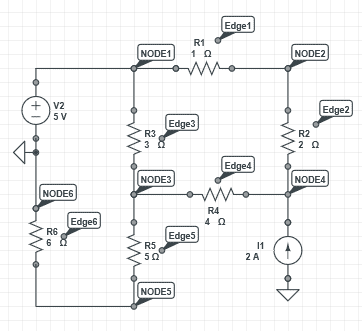This should be ok correct? With the ground at node 6 acting as a return to the current source.

The only problem is that I don't think this changes my equations and MATLAB will still give me an error saying that the matrix is singular and still through the error. I'm not sure how to get around that. What can I do?

Thanks for all of your help!

Gold Member
...I don't think this changes my equations...
So run it in the simulator. If it runs or gives a different error message then the equations changed.
Let us know the results.

(I'll take bets on this.{Well, probably not on this site.})

YoshiMoshi
Ya that's the problem though. I got it to run in the simulator however my MATLAB equations stay the same and I still get the singularity matrix problem. How can I get MATLAB to give me the correct answer?
View attachment 99227
View attachment 99228
View attachment 99229
View attachment 99230
View attachment 99231
putting my equations into MATLAB provides with the following answer as well as a warning that the matrix is singular
View attachment 99232
MATLAB commands

>> N=[-1,1,0,0,0,0;0,-1,0,1,0,0;-1,0,1,0,0,0;0,0,1,-1,0,0;0,0,-1,0,1,0;0,0,0,0,-1,1];
>> R=[1,0,0,0,0,0;0,2,0,0,0,0;0,0,3,0,0,0;0,0,0,4,0,0;0,0,0,0,5,0;0,0,0,0,0,6];
>> A=[0,0,0,0,0,0;0,0,0,0,0,0;0,0,0,0,0,0;0,0,0,0,0,0;0,0,0,0,0,0;0,0,0,0,0,0];
>> E=[0;0;0;0;0;5];
>> I=[0;0;0;0;3;0];
>> inv([R,N;N',A])*[I;E]
Warning: Matrix is close to singular or badly
scaled. Results may be inaccurate. RCOND =
2.312965e-18.

ans =

1.0e+16 *

0
0
0
0
0.0000
0.0000
4.5036
4.5036
4.5036
4.5036
4.5036
4.5036

and when I try to take the determinant of the matrix [R,N;N',A] I get

-1.1102e-15

I have to restart my simulator I'll edit this post with what it gives me. I just don't understand what's wrong with MATLAB telling me that it's singular and throwing me that error?

EDIT:

My simulator says
V1 = 5 V
V2 = 6.244 V
V3 = 5.710 V
V4 = 8.733 V
V5 = 3.115 V
V6 = 0.000 V
I_R1 = 1.244 A
I_R2 = 1.244 A
I_R3 = 236.6 mA
I_R4 = 755.7 mA
I_R5 = 519.1 mA
I_R6 = 519.1 mA

But I can't seem to figure out why MATLAB is giving me an error and not allow me to get the correct answer =( what do I do.

Last edited:
Gold Member
I'm confused. You change a circuit and the describing equations did not change? How can that be? The equations describe the circuit topology.

Lets see if @The Electrician can come back and handle the MATLAB stuff.

YoshiMoshi
Yeah exactly. That's why I was confused about. I originally posted this in the calculus and beyond section because my problem was with the linear algebra part and not necessarily the electrical engineering part. Yeah I don't think it changes.

The incidence matrix N remains the same. Right?
The resistance matrix definitely stays the same.
I and E stay the same.

I guess for the incidence matrix, once I add in the ground at node 6, the current on edge 6 (R6) is still leaving node 5 and entering node 6 right? So yeah I don't think it changes.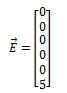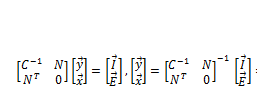I'm strugglign with the linear algebra part =(

Gold Member
Unfortunately it's been too many decades since I've done any matrix algebra so I can't be of much help.
I did notice your node numbering changed between posts #5 & #6 though. If you haven't yet, how about writing the MATLAB stuff from scratch again from your current schematic? We all get blind to our own errors after about the third pass.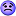YoshiMoshi
Oh dear your right! Thanks for catching that. Let me see for my current schematic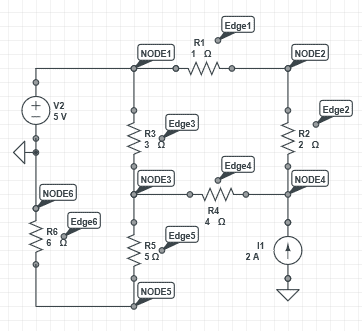it appears that the incidence matrix is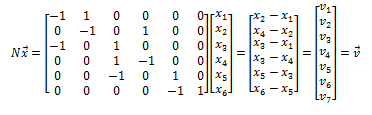I think my incidence matrix was right for my current schematic in this post! Was wrong for post in 5 Let me retry in MATLAB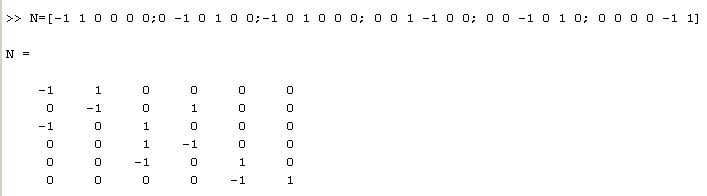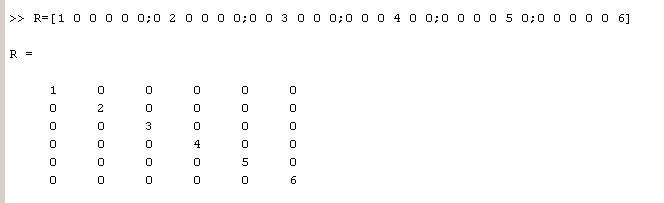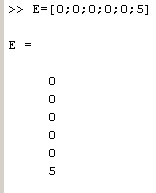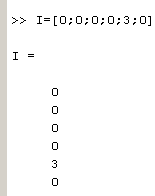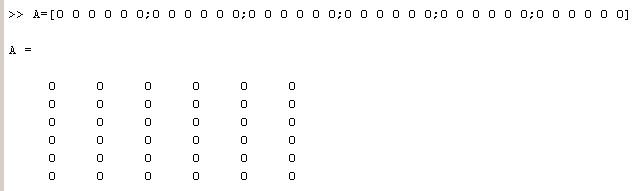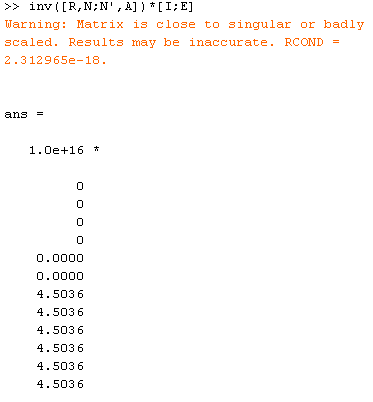=( I'm stuck

Staff Emeritus
You started this thread with a voltage source and two current sources. Now you have "updated" it by removing one current source, apparently. Is the circuit now as you desire it?

YoshiMoshi
Yeah it is and it's runniung in my simulator, my only concern is MATLAB is not providing me an answer. I seem to be struggling more so with the linear algebra part now. I'm not exactly sure what I'm doing wrong =(. Thanks for any help.

Staff Emeritus
Homework Helper
I don't think the problem is with the linear algebra. Your problem seems to be in deriving the correct equations that correspond to this circuit. The fact that you can change the circuit and yet not change the equations should be a giant red flag to you that your method is wrong.

Look at what you wrote here:
View attachment 99235
How can it possibly be correct? You have a six-component vector set equal to a seven-component vector.

Can you define for us what the x's and y's stand for? From a dimensional analysis point of view, something is amiss. It looks like the y's are voltages, yet you're multiplying them by R and getting currents. That is, your version of Ohm's law is I=VR.

YoshiMoshi
Yea your right about that. Thanks for catching that. It's a typo. It's supposed to be: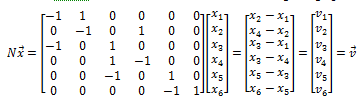That does seem to be problematic. But I'm not exactly sure what would change in the equations. The incidence matrix N and six columns and six rows. The first column represents node 1, the second column represents node 2, and so forth. Row 1 represents edge 1, row 2 represents edge 2, and so forth. I populate the incidence matrix N by looking at each edge and if crrent is leaving the node I put a negative one, and if it's entering the node I put a positive one.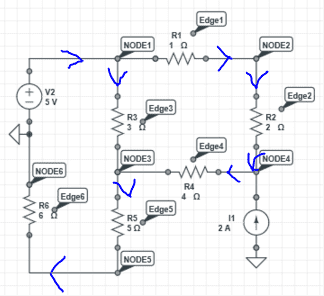The vector V is the voltage across each resistor. At least this is how I was taught but to me it looks like the opposite of the voltage drop. Like I have the following
X_2 - X_1 = V_1 when in my opinion X_1 - X_2 = V_1. But this is how we were taught, not sure if it's wrong?

The resistance matrix R is just a diagonal matrix, where column one row one represents R_1, column 2 row 2 represents R_2 and so forth.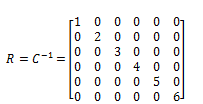HW solutions solved a similar problem in a similar way but the final calculation was never done. My text book doesn't seem to have a resistive circuit example.

Yeah
X_1 = nodal voltage at node 1
X_2 = nodal voltage at node 2
X_3 = nodal voltage at node 3
.
.
.

Y_1 = current going across edge 1 (R_1)
Y_2 = current going across edge 2 (R_2)
.
.
.

Also C^(-1) = R
so I use the following relation
The matrix I represents the applied currents to the circuit. Since I only have one current source at node 4 I get the following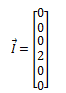looks like I had it wrong before by accident.
The matrix E represents the applied voltage sources in the circuit which is only at node 6 so E is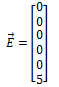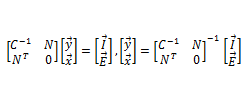I try re-entering this into MATLAB and create the zero matrix A to help.
>> N=[-1 1 0 0 0 0;0 -1 0 1 0 0;-1 0 1 0 0 0;0 0 1 -1 0 0;0 0 -1 0 1 0;0 0 0 0 -1 1]

N =

-1 1 0 0 0 0
0 -1 0 1 0 0
-1 0 1 0 0 0
0 0 1 -1 0 0
0 0 -1 0 1 0
0 0 0 0 -1 1

>> R=[1 0 0 0 0 0;0 2 0 0 0 0;0 0 3 0 0 0;0 0 0 4 0 0;0 0 0 0 5 0;0 0 0 0 0 6]

R =

1 0 0 0 0 0
0 2 0 0 0 0
0 0 3 0 0 0
0 0 0 4 0 0
0 0 0 0 5 0
0 0 0 0 0 6

>> I=[0;0;0;2;0;0]

I =

0
0
0
2
0
0

>> E=[0;0;0;0;0;5]

E =

0
0
0
0
0
5

>> A=[0 0 0 0 0 0;0 0 0 0 0 0;0 0 0 0 0 0;0 0 0 0 0 0;0 0 0 0 0 0;0 0 0 0 0 0]

A =

0 0 0 0 0 0
0 0 0 0 0 0
0 0 0 0 0 0
0 0 0 0 0 0
0 0 0 0 0 0
0 0 0 0 0 0

>> inv([R N;N' A])*[I;E]
Warning: Matrix is close to singular or badly
scaled. Results may be inaccurate. RCOND =
2.312965e-18.

ans =

1.0e+16 *

0.0000
0.0000
-0.0000
0.0000
0.0000
0.0000
4.5036
4.5036
4.5036
4.5036
4.5036
4.5036

to solve for the matrix [y;x]. The problem is that when I plug this into MATLAB I get the error and I don't why =(. Thanks for any help.